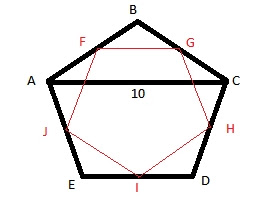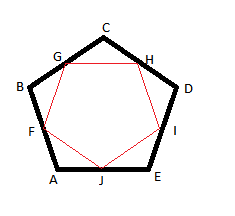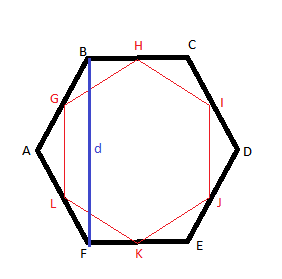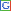## Friday, January 20, 2012

### I Have An Answer To My Question And A Solution To My Puzzle!

In my previous post, I talked about a puzzle that was triggered by a geometry problem that my daughter brought home from school. The puzzle is quite simple, and involves midpoint polygons. These are inscribed polygons that are derived by joining the midpoints of the sides of the exterior polygons. The puzzle was to find the ratio of the perimeter of the midpoint polygon to the perimeter of the outer polygon as a function of the number of sides of the polygon.Last week I stopped at hexagons because my techniques were not good enough to allow me to calculate the perimeter of a midpoint heptagon given a regular exterior heptagon of unit sides. But, I kept thinking about the puzzle on and off, and have now come up with a solution that should work for any regular polygon. In this post, I am going to explain how my method works, and present some results of my investigations.

The good news is that it is possible to express the ratio in terms of the number of sides for any regular polygon. The bad news is that the formula looks quite ugly and complicated. All the simple formulas like E=mc2 are already taken!The secret to working out the ratio starts with drawing a diagonal that connects two alternate vertices of the exterior polygon. By alternate vertices, I mean two vertices that are separated by one intermediate vertex. In the figure to the left, consider segments AB and BC to be part of the exterior polygon (I have not drawn the rest of the polygon because I want to derive a formula that applies to polygons with any number of sides. So, my figure only shows two sides of a polygon with an arbitrary number of sides). They are equal to each other because the polygon is regular. Let us assume they are each 1 unit in length.

Segment AC is a diagonal that connects two alternate vertices of the exterior polygon (B is the intermediate vertex that is skipped over by the diagonal). ABC is now an isosceles triangle. Angle ABC is an interior angle of the polygon, and it is a function of the number of sides the polygon has. In particular, we know that angle ABC is equal to (n-2)*180/n degrees, where n is the number of sides of the regular polygon. In the figure, I have represented this angle by x.

We also know that angles BCA and BAC are equal, and because they are part of triangle ABC, we know that each is equal to (180–x)/2. Since x = (n-2)*180/n degrees, we can substitute that in the expression above, and get the measure of angles BAC and BCA to be 360/2n degrees. In the figure, I have represented this angle by y.

Surprisingly, that is all the information we need to get the ratio we are after. The first step in calculating the ratio is the calculation of the length of segment AC. Why? Because, we know from my previous post that each side of the midpoint polygon is half the length of the diagonal that connects alternate vertices of the exterior polygon.

So, how do we go about calculating the length of segment AC? We apply the law of sines to triangle ABC to calculate the length of segment AC. We know that angle x is opposite to the segment AC whose length we want, and angle y is opposite the segment AB whose length is 1. Thus, 1/sin(x) = AC/sin(y). Therefore, AC = sin(y)/sin(x).

At this point, we can express x and y in terms of n, and we will have AC in terms of n. Divide the length of AC by 2, and that is the ratio we are after (the length of one of the sides of the midpoint polygon). Doing these substitutions, we have:

AC = sin(360/2n)/sin((n-2)*180/n)

Therefore, the ratio of the perimeter of the midpoint polygon to the perimeter of the regular exterior polygon (which is half of AC) would be:

R = sin(360/2n)/(2*sin((n-2)*180/n))

Well, it may not be pretty, or roll off the tongue quite like E=mc2, but it is a function of n, the number of sides of the polygon, and that is what we started out seeking. So, pretty or not, we have achieved what we wanted to achieve, and anyway, half the fun of getting there is the traveling itself, right? So what if the destination was not stunning, the route was plenty scenic, right?!

Now, how do I know this is correct? Below is a table with the value of R calculated for regular polygons with different number of sides. The first 3 lines correspond to polygons that we dealt with in the previous post (squares, pentagons and hexagons). I had to start with squares because triangles do not have diagonals (unless you consider each side to be a diagonal also). As you can see, the value of the ratio as calculated using the above formula is identical to the value of the ratio which we derived using other methods in the previous post.

 Number of Sides (n) Interior Angle ABC (x) (in degrees) Angle with Diagonal (y) (in degrees) Length of Diagonal AC Ratio of Perimeters (R) 4 90 45 1.414213562 0.707106781 5 108 36 1.618033989 0.809016994 6 120 30 1.732050808 0.866025404 7 128.5714286 25.71428571 1.801937736 0.900968868 8 135 22.5 1.847759065 0.923879533 9 140 20 1.879385242 0.939692621 10 144 18 1.902113033 0.951056516 100 176.4 1.8 1.999013121 0.99950656 1,000 179.64 0.18 1.99999013 0.999995065 10,000 179.964 0.018 1.999999901 0.999999951 1,000,000 179.99964 0.00018 2 1

It is interesting to note that, as predicted, the ratio does approach 1 as the number of sides goes up. I have added rows for 100, 1000, 10000 and 1000000 sides to the table above just to illustrate how the ratio gets closer to 1 as the number of sides goes up. There are so many 9's in the answers for the polygon with a million sides that I decided to do away with them, and put down the answer rounded to a billionth!

By the way, a polygon with 100 sides is called a hectogon. A polygon with 1,000 sides is called a chiliagon, one with 10,000 sides is called a myriagon, and one with a million (1,000,000) sides is called a megagon!

Since we know that the ratio of the area of an inscribed midpoint polygon to the area of the exterior regular polygon is the square of the ratio of perimeters, you can just take the numbers from the table in this post and square them to get the ratios for the areas without having to explicitly calculate the areas of either the exterior polygons or the inscribed midpoint polygons.

There exist formulas for the area of a regular polygon based on the number of sides, and the length of each side, so if you are curious, you can calculate the area of the exterior polygon using such a formula, calculate the area of the midpoint polygon using the ratio in the table to compute the length of each side, and then calculate the ratio of the areas. The amazing thing about mathematics is that you can derive general results (such as: the ratio of areas is the square of the ratio of the perimeters), and after that you don't have to do all the hard work involved in calculating specific results. But the tools exist to do so if you want to.

Well, I consider this another example of using very simple, well-known concepts, and stringing them together in just the right order to derive something a little more interesting and complicated. It is what makes and keeps mathematics so interesting and challenging. And hopefully, it will keep our brains young and fresh! Good luck in your mathematical explorations!!

## Thursday, January 12, 2012

### An Interesting Geometrical Puzzle

My daughter recently brought home a puzzle that had me scratching my head for a while before being able to solve it. Actually, the solution was quite simple once I figured it out, but it spawned off a different puzzle in my head that may be a little trickier. In any case, I have not figured out a solution to the spawned-off puzzle yet, but let me not get ahead of myself. Let me first tell you about the puzzle that my daughter brought home.You are given a regular pentagon, ABCDE (regular in this context means that all the sides are of equal length, and all the interior angles are equal to 108 degrees). You are also given the fact that the diagonal AC of this pentagon is 10 units long. Please refer to the figure on the left.

Now, inside this pentagon is another pentagon formed by joining the midpoints of each of the sides of the original pentagon. Call this inscribed pentagon FGHIJ. The puzzle is to use all this information to find the perimeter of the pentagon FGHIJ.

Initially, for some reason, I thought the solution would consist of finding the perimeter of ABCDE first and then somehow using that along with the length of AC to derive the perimeter of FGHIJ. That is what had me floundering for a few minutes. In fact, I even looked up the Wikipedia article on pentagons for some inspiration.

But the solution is actually much simpler (obviously, the fact that this puzzle was given to a middle-school student as an assignment is a big hint that this is not the geometric equivalent of Fermat's last theorem!). It is simply sufficient to realize that the diagonal creates a triangle (ABC in this case), and the line segment FG connects the midpoints of two of the sides of this triangle.

You can then apply the midpoint theorem on this triangle to conclude that side FG must be equal to 5 units in length because AC is 10 units long. And because FGHIJ is a regular pentagon, being the midpoint pentagon of a regular pentagon, its perimeter must be 5*5 = 25 units.

But, obviously, half the fun of solving a mathematical problem is coming up with related puzzles and questions. In particular, the puzzle I am now stuck with is as follows: what is the ratio of perimeters of midpoint polygons to the perimeters of the polygons they are inscribed in as a function of the number of sides of the polygon? Similarly, what is the ratio of areas of midpoint polygons to the perimeters of the polygons they are inscribed in as a function of the number of sides of the polygon?

Note that the polygons formed by joining the midpoints of the sides of a given polygon are called midpoint polygons, and have been studied by mathematicians in some detail. In particular midpoint polygons constructed from regular polygons are also regular, and are geometrically similar to the exterior polygon.Let us take a regular triangle (also known as an equilateral triangle) first. Connecting the midpoints of the three sides to create an inscribed midpoint triangle inside the original triangle gives us a triangle with half the perimeter as the original. Note that in the figure below, triangle ADF is also equilateral, and since AD and AF are one half of the length of AB, DF is one-half of the length of AB. And since DE, EF and DF have the same length (each equal to half the length of the sides of the original triangle ABC), the new triangle has half the perimeter as the original triangle.

Also note that the original triangle ABC has been divided into 4 equilateral triangles of equal area by the addition of the inscribed triangle DEF. Thus, the area of triangle DEF is one quarter of the area of the original triangle ABC. Thus, the ratio of perimeters is 1/2, and the ratio of areas is 1/4.Moving on to a square, which is a regular quadrilateral, we can use the pythagorean theorem on triangle EBF to figure out that the length of EF is equal to (length of AB)/sqrt(2). And the area of EFGH is one-half of the area of ABCD. Thus, the ratio of perimeters is 1/sqrt(2), and the ratio of areas is 1/2.The situation becomes more complicated in the case of a pentagon. In fact, it is not easy to figure out what the length of each side of an inscribed pentagon is if you know just the lengths of the sides of the original pentagon. But my research into regular pentagons on the Wikipedia site did have a positive side-effect: it turns out that the diagonals of a regular pentagon are in golden ratio to its side. In other words, if the length of a side is 1 unit, the length of a diagonal is eaual to the golden ratio. The value of golden ratio is 1.6180339887498948482045868343656.

From my solution of the puzzle which started this entire exploration, we also know that the length of each side of the inscribed pentagon is one half of the length of the diagonal. Thus, if the length of each original side is 1, then each diagonal is 1.6180339887498948482045868343656 units long, and the length of each inscribed side is 1.6180339887498948482045868343656/2 units long. Thus, the ratio of perimeters is 1.6180339887498948482045868343656/2.

The same Wikipedia article also tells me that the area of a regular pentagon with sides of length t is 1.720477401*t^2. Thus, the area of the inscribed pentagon would be 1.720477401*(1.6180339887498948482045868343656*t/2)^2. What we are interested in is the ratio of the areas, not the actual areas themselves. And that would simply be (1.6180339887498948482045868343656/2)^2.What about a hexagon? Well, the analysis is a little more complicated in the case of a hexagon. First of all there are two formulas for the area of a hexagon that we can use to derive the length of a diagonal of the hexagon that connects two alternate vertices of the hexagon (note that a hexagon also has three diagonals that connect opposite vertices, and these diagonals are twice the length of each side. They are also longer than the diagonals we are interested in). You can find these formulas in the wikipedia article on hexagons.

The first formula for area is: area = 2.598076211*t^2. The second formula for area is: area = 1.5*d*t. Interestingly, d is the length of the diagonal connecting alternate vertices of the hexagon (it is the height of the hexagon when it is resting on one of the sides as its base). Using these two formulas, since the area of a hexagon is a single number, we can say that 2.598076211*t^2 = 1.5*d*t. From this, we can derive the value of d to be 2.598076211*t/1.5.

At this point, we can then use the midpoint theorem to say that each side of an inscribed hexagon would be one-half the length of each of these diagonals. Thus the ratio of perimeters would be 2.598076211/3. And the ratio of areas would be the square of that number since the area is directly proportional to the square of the length of a side.

So, that is as far as I have gotten so far. The area formula for heptagons does not allow me to calculate the length of a diagonal connecting alternate vertices of a heptagon. So, I can not calculate the length of a side of an inscribed midpoint heptagon either. You could say, I am stuck!

In table form, one can express this as below:

 Number of Sides Ratio of perimeter of midpoint polygon to perimeter of exterior polygon Ratio of area of midpoint polygon to area of exterior polygon 3 0.50 0.25 4 0.70710678118654752440084436210485 0.50 5 0.8090169943749474241022934171828 0.65450849718747371205114670859138 6 0.86602540366666666666666666666667 0.75

Pretty basic observations follow from this.
• For regular polygons, area seems to be always proportional to the square of the length of a side. Thus, the ratio of areas of inscribed midpoint polygons to exterior polygons would be the square of the ratio of perimeters.
• The ratio of perimeters (and thus the areas) approaches 1, and will be 1 in the asymptotic case of a polygon with infinite number of sides (one could argue that the inscribed midpoint circle of a given circle is coincident with the given circle, and thus the ratio of perimeters as well as areas is indeed 1).
• Since the ratio of areas is the square of the ratio of perimeters, and the ratio of perimeters is less than 1.0, the ratio of areas is always less than the ratio of perimeters.

These observations though, do not tell me the answer to the question I started with: what is the ratio of the perimeter of an inscribed midpoint polygon to the perimeter of the exterior polygon? Is it possible to find an expression that tells me the value of this ratio for any type of polygon (obviously, such an expression would be a function of the number of sides of the polygon)? Any ideas? I would love to hear your thoughts on how to proceed with this investigation. Thank you, and good luck!

## Wednesday, January 11, 2012

### Anti-Reflective Coatings Have Come A Long Way

Anti-reflective coatings are used on optical lenses to increase the collecting of light, and to prevent stray light from bouncing around inside systems with multiple lenses. They have been around for a while now, having been invented in Germany in the 1930's. Anti-reflective coatings on corrective glasses make them look better, and also reduce glare, especially in high-contrast situations like night-time driving.

When I bought my daughter's latest pair of eyeglasses, I decided to go with Zenni's latest anti-reflective coating on the lenses. This coating is the latest generation, and is technically advanced. Not only does it reduce glare and make the glasses less likely to stand out in photographs, but it also boasts several advanced features.

This new generation of AR coatings is hydrophobic and oleophobic. This means that the coating repels not only water, but also oils. Repelling oil is significant because most smudges, fingerprints, etc., on lenses are caused by the oils on your hands and fingers. By repelling most oils, the oleophobic lenses stay cleaner longer, and are much easier to clean.

The differences between her old pair of glasses and the new pair of glasses has made a believer out of me as far as this coating is concerned. My daughter gets crystal clear vision without having to deal with glare from table lights, her computer screen, overhead lights, etc. Since more light passes through the lenses instead of being reflected away, things appear brighter, and with better contrast. The lenses have been much easier to clean and keep clear of dirt, smudges and fingerprints. And, best of all, she likes the fact that she does not have remove her glasses before posing for photographs!

## Content From TheFreeDictionary.com

In the News

Article of the Day

This Day in History

Today's Birthday

Quote of the Day

Word of the Day

Match Up
 Match each word in the left column with its synonym on the right. When finished, click Answer to see the results. Good luck!

Hangman

Spelling Beedifficulty level:
score: -